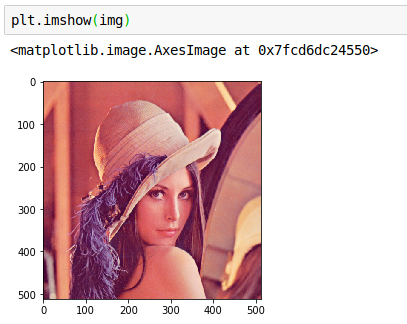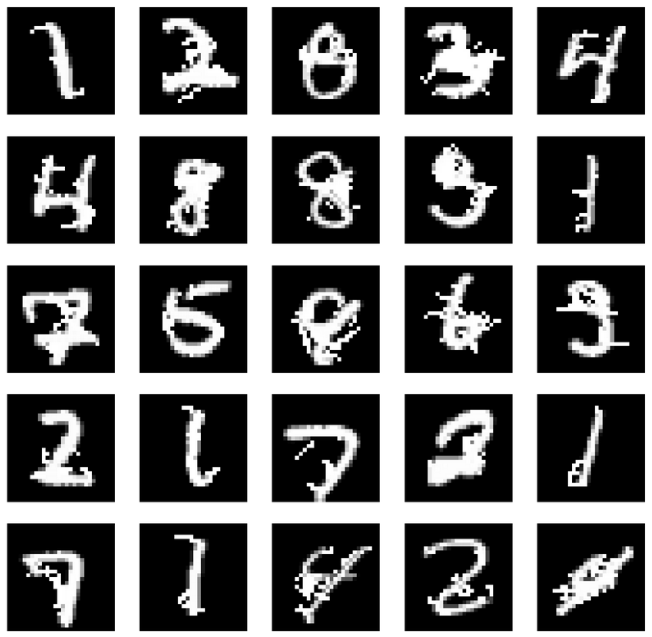# Python图像处理基本操作

• OpenCV（Open Source Computer Vision Library）
• PIL（Python Imaging Library）
• skimage（scikit-image）

*如下代码的实验环境为Jupyter Notebook.

## 1. OpenCV

OpenCV是图像处理中最强大的一个库，它的源代码是由C\C++写成的，所以原版的OpenCV可以与C、C++无缝结合。Python版的OpenCV主要依赖于cv2这个包来实现。

import cv2
import numpy as np

print(img.shape)
print(type(img))

(512, 512, 3)
<class 'numpy.ndarray'>


### 1.2  imshow()

cv2.imshow('Lena',img)
cv2.waitKey(0)
cv2.destroyAllWindows()

### 1.3  imwrite()

cv2.imwrite('lena.jpg',img)

## 2. PIL

Python里面自带一个PIL（python images library), 但这个库现在已经停止更新了，所以使用Pillow, 它是由PIL发展而来的。

### 1.1  open()

from PIL import Image
import numpy as np

img = Image.open('Lena.png')
print(img.size)
print(img.mode)
print(type(img))

(512, 512)
RGB
<class 'PIL.PngImagePlugin.PngImageFile'>


### 1.2 show()

img.show()

import matplotlib.pyplot as plt
%matplotlib inline

plt.imshow(img)

inline一句的作用是在Jupyter Notebook的网页中直接绘图，即不会单独弹出绘图窗口，上述代码的执行结果如下：plt.axis('off')
plt.imshow(img)

fig=plt.figure(figsize=(28, 28))
columns = 5
rows = 5
for i in range(1, columns*rows +1):
plt.imshow(img, cmap='gray')
plt.axis('off')### 1.3  save()

img.save('lena2.jpg')

im = Image.fromarray(np.uint8(data_jsma_0*255))
im.save("000.png")

## 3. skimage

from skimage import io
import numpy as np

print(img.shape) # numpy矩阵，(h,w,c)

print(type(img))

(512, 512, 3)
<class 'numpy.ndarray'>


### 1.2  img_as_ubyte()

print(np.max(adv_test_cw0))

print(np.min(byte_adv_test_cw0))

0.9997719
0.00054621696

255
0

【全文完】

©️2019 CSDN 皮肤主题: 精致技术 设计师: CSDN官方博客